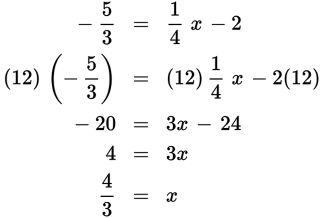# SAT Math Multiple Choice Question 439: Answer and Explanation

### Test Information

Question: 439

4. Given the function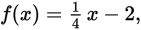what domain value corresponds to a range value of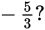• A.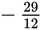• B.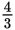• C.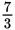• D.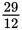Explanation:

B

Difficulty: Medium

Category: Passport to Advanced Math / Functions

Strategic Advice: Don't answer this question too quickly—you may be tempted to substitute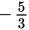for x, butis the output (range), not the input (domain).

Getting to the Answer: The given range value is an output value, so substitutefor f(x) and use inverse operations to solve for x, which gives you the corresponding domain value. Start by multiplying the equation by the greatest common multiple of 3 and 4, which is 12, in order to clear the fractions.